# 知名网站热门排序算法分析

## Hacker News

Hacker News 是一家关于计算机黑客和创业公司的社会化新闻网站，由 Paul Graham 的创业孵化器 Y Combinator 创建。与其它社会化新闻网站不同的是 Hacker News 没有踩或反对一条提交新闻的选项（不过评论还是可以被有足够 Karma 的用户投反对票，或是投支持票）；只可以赞或是完全不投票。简而言之，Hacker News 允许提交任何可以被理解为“任何满足人们求知欲”的新闻。• 点赞数（每个新闻标题前面有一个向上的三角形，如果你觉得这个内容很好，就点击一下，投上一票）
• 距离发布的时间
• 评论量

• 点赞数：相同发帖时间，投票数越高，分值越高
• 距离发布时间：相同投票数，距离发帖时间越近，分值越高

$$score = \frac{P}{t}$$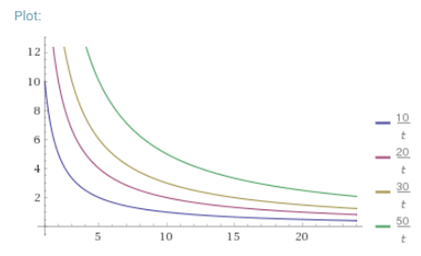$$score = \frac{P-1}{(t+2)^{1.5}}$$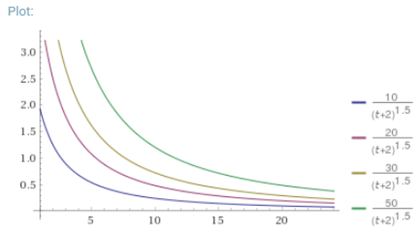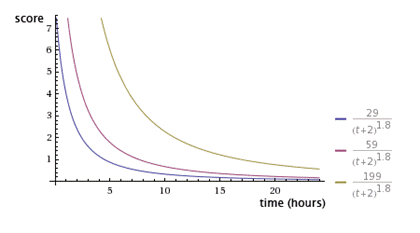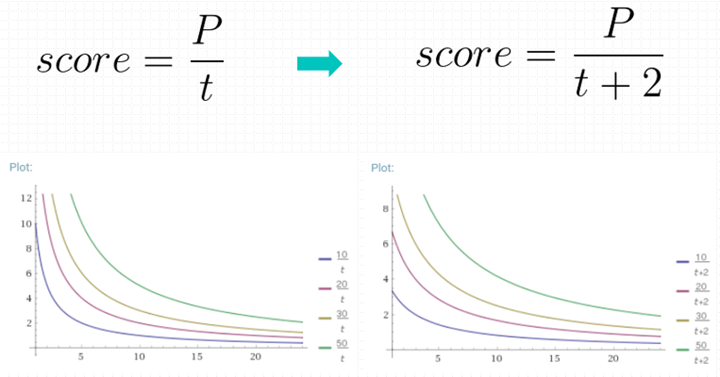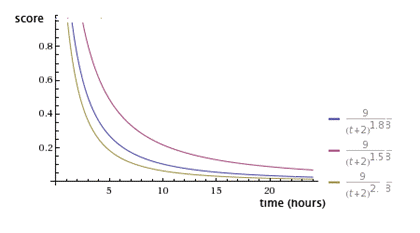$$score = \sum (orderCount * \alpha ^t)$$

• 1周后的权重降为5，则$\alpha$ ^7=0.5，$\alpha$≈0.905724
• 2周后的权重降为5，则$\alpha$ ^14=0.5，$\alpha$≈0.951695
• 3周后的权重降为5，则$\alpha$ ^21=0.5，$\alpha$≈0.967532
• 4周后的权重降为5，则$\alpha$ ^28=0.5，$\alpha$≈0.975549

import datetime
import pandas as pd
ALPHA = 0.97

def get_score(order_count, days):
return order_count * pow(ALPHA, days)

df.columns = ['id', 'item_id', 'create_date']
df['days'] = (datetime.datetime.now() - df.create_date).astype('timedelta64[D]')
df_s = df.groupby(['item_id', 'days']).size().reset_index(name='order_count')
df_s["score"] = get_score(df_s.order_count, df_s.days)
df_m = df_s.groupby("item_id").score.sum().reset_index(name='score')
df_m.to_cvs("result.csv")


• 通常在计算时可以取30天的数据，原因是某个<1数字的30次方已经非常小了，计算的意义不大
• 以上的计算针对的是有热度的内容或产品，如果部分内容不随时间衰减，使用上诉公式不影响

SELECT itemid
, SUM(order_count * pow(0.9, recency)) AS score
FROM (
SELECT mo.itemid, datediff(current_date(), mo.createdate) AS recency
, COUNT(DISTINCT mo.memberid) AS order_count
FROM db.memberorder mo
GROUP BY mo.itemid, datediff(current_date(), mo.createdate)
) t
GROUP BY itemid
ORDER BY score DESC
LIMIT 100


## Reddit文章

Reddit与Hacker News类似，也是一个社会化新闻网站，Reddit对文章和评论使用了不同的排名算法，这里介绍的排序规则主要针对的是文章排序。Reddit与Hacker News有很大的不同点就是，Hacker News文章标题前面只有一个向上的小箭头，即只能投赞成票，而Reddit的每个文章标题前会有两个箭头，即一个向上，一个像下。分别代表“赞成”与“反对”。 Reddit已经把他们的所有源代码进行了公开，具体涉及到排序部分的代码如下：https://github.com/reddit/reddit/blob/master/r2/r2/lib/db/_sorts.pyx。由于此部分代码是使用Python的C语言扩展来写，下面是用Python重写的代码：

from datetime import datetime
from math import log

epoch = datetime(1970, 1, 1)

def epoch_seconds(date):
""" Returns the number of seconds from the epoch to date. """
td = date - epoch
return td.days * 86400 + td.seconds + (float(td.microseconds) / 1000000)

def up_down(ups, downs):
return ups - downs

def hot(ups, downs, date):
""" The hot formula. Should match the equivalent function in postgres. """
z = up_down(ups, downs)
order = log(max(abs(z), 1), 10)
sign = 1 if z > 0 else -1 if z < 0 else 0
seconds = epoch_seconds(date) - 1134028003
return round(order + sign * seconds / 45000, 7)


$$score = \log_{10}z+\frac{yt_s}{45000}$$

• z：获得的投票数的绝对值（赞成-反对），当=0时，z=1
• y：投票方向，投票数＞0时，y=1；y＝0时，y=0；＜0时，y=-1
• $t_s$：发布时间距离2005年12 月 8 日07:46:43的秒数

t = 发表时间 – 2005 年 12 月 8 日7:46:43

• 发表时间对排名有很大影响，该算法使得新的话题比旧的话题排名靠前
• 话题的得分不会因为时间的流失而减少，但是新的话题会比旧的话题得分高。这与 Hacker New 的算法不同 （随着时间的发展降低话题的得分）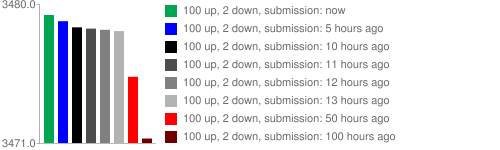x = 赞成票-反对票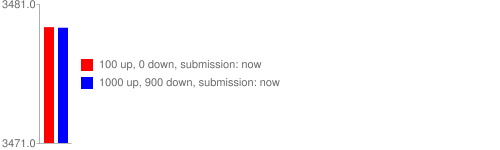$$\log_{10}z$$

Reddit 的热排序算法使用了对数函数来衡量前面的投票与其他投票的差距使其前十个好评和之后的100个，1000个投票有相同的权重。 参见下面的图：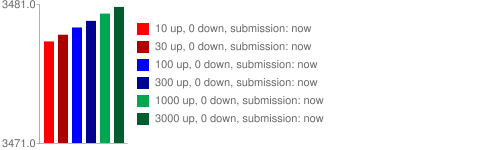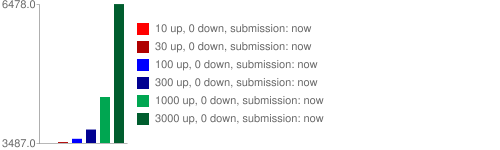Reddit敢于如此消弱投票的作用，其实与其庞大的流量和用户参与度相关。如果没有以上因素算法很难实现很好的推荐。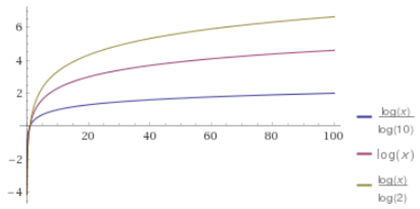$$\frac{yt_s}{45000}$$

y 的作用是用来产生正分和负分。当赞成票超过反对票时，得分为正；当赞成票少于反对票时，得分为负；当两者相等，得分为0。这就保证了得到大量净赞成票的文章，会排在前列；得到大量净反对票的文章，会排在最后。投票对于总分的贡献不大，但是当投票的意见倾向发生变化时（由正面评价转向负面评价），投票对于总分的作用却是决定性（Y的取值）。

## Reddit评论

• A:好评550;　差评450
• B:好评60；差评40
• C:好评1；差评0
• D:好评9，差评1

• 每个用户的投票都是独立事件。
• 用户只有两个选择，要么投好评，要么投差评。
• 如果投票总人数为n，其中好评为k，那么好评率p就等于k/n。

p越大，就代表这个项目的好评比例越高，越应该排在前面。但是，p的可信性，取决于有多少人投票，如果样本太小，p就不可信。由于p服从”两项分布”，因此我们可以计算出p的置信区间。所谓“置信区间”，就是说，以某个概率而言，p会落在的那个区间。比如，某个产品的好评率是 80%，但是这个值不一定可信。根据统计学，我们只能说，有 95% 的把握可以断定，好评率在 75% 到 85% 之间，即置信区间是[75%， 85%]。

• 计算每个评论的“好评率”
• 计算每个“好评率”的置信区间（以 95% 的概率）。
• 根据置信区间的下限值，进行排名。这个值越大，排名就越高。

• A有 8 张赞成票，2张反对票
• B有 80 张赞成票，20张反对

• A的置信区间（假定[70%， 90%]）
• B的置信区间（假定[75%， 85%]）

B的置信区间的下限值（75%）会比A（70%）大，所以B应该排在A前面。置信区间的实质，就是进行可信度的修正，弥补样本量过小的影响。如果样本多，就说明比较可信，不需要很大的修正，所以置信区间会比较窄，下限值会比较大；如果样本少，就说明不一定可信，必须进行较大的修正，所以置信区间会比较宽，下限值会比较小。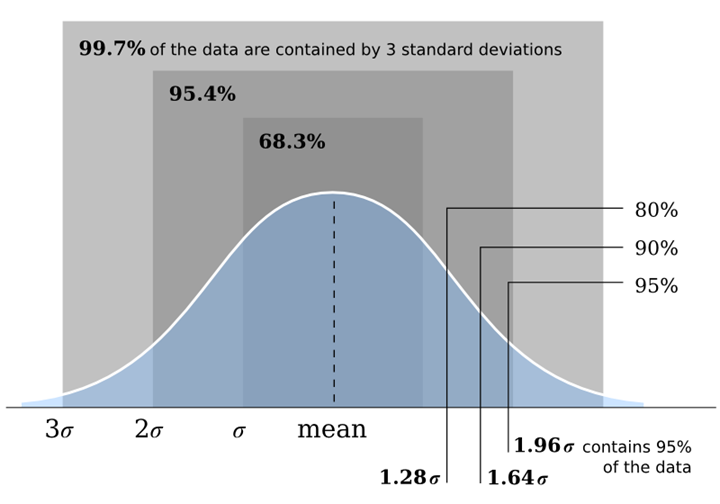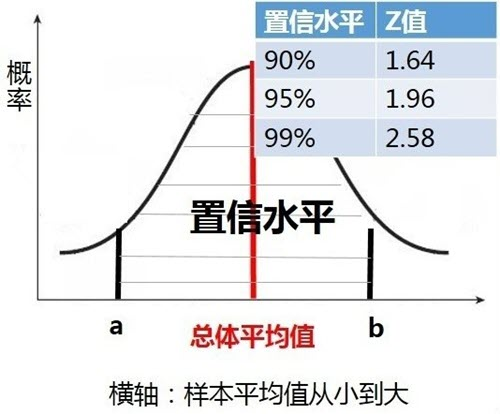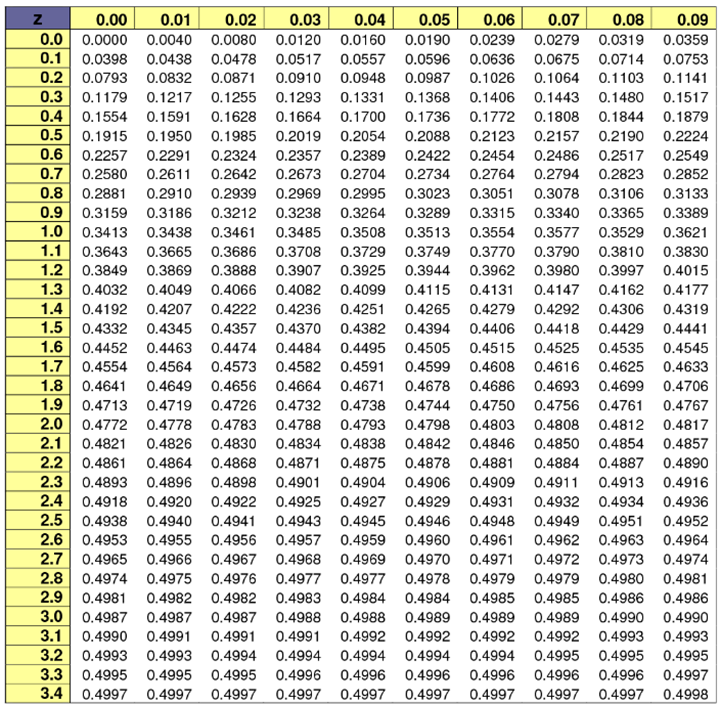• a = 总体平均值$\mu$ – 标准分位z * 标准误差SE
• b = 总体平均值$\mu$ + 标准分位z * 标准误差SE

$$SE=\frac{S}{\sqrt{n}}$$

• SE：标准误差
• s：样本标准差
• n：样本大小

1927年，美国数学家 Edwin Bidwell Wilson 提出了一个修正公式，被称为威尔逊区间，很好地解决了小样本的准确性问题。Reddit 目前使用的是评论算法就是基于威尔逊得分区间 (Wilson score interval)。具体代码片段可从开放的源代码中找到，将其转化成Python代码后：

from math import sqrt

def _confidence(ups, downs):
n = ups + downs

if n == 0:
return 0

z = 1.0 #1.0 = 85%, 1.6 = 95%
phat = float(ups) / n
return (phat+z*z/(2*n)-z*sqrt((phat*(1-phat)+z*z/(4*n))/n))/(1+z*z/n)

def confidence(ups, downs):
if ups + downs == 0:
return 0
else:
return _confidence(ups, downs)


$$\frac{\hat{p}+\frac{1}{2n}z^2 \pm z\sqrt{\frac{\hat{p}(1-\hat{p})}{n}+\frac{z^2}{4n^2}}}{1+\frac{1}{n}z^2}$$

• p 是好评率
• n 是总投票数
• $z$表示对应某个置信水平的z统计量，这是一个常数，可以通过查表得到。一般情况下，在 95% 的置信水平下，z统计量的值为96。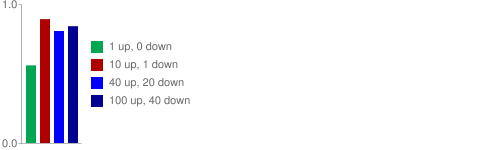• 垃圾邮件检测：看到这个内容并将它标记成垃圾邮件的百分比有多少？
• 创建精华列表：看到这个内容并将它加星标件的百分比有多少？
• 创建最受欢应列表：看到这个内容并将它转发给朋友的百分比有多少？

## IMDB

IMDB.COM是目前互联网上最为权威、系统、全面的电影资料网站，里面包括了几乎所有的电影，以及1982 年以后的电视剧集。 它所特有的电影评分系统深受影迷的欢迎，注册的用户可以给任何一部影片打分并加以评述，而网站又会根据影片所得平均分、选票的数目等计算得出影片的加权平均分并以此进行TOP250（最佳250部影片）和Bottom100（最差100部影片）的排行。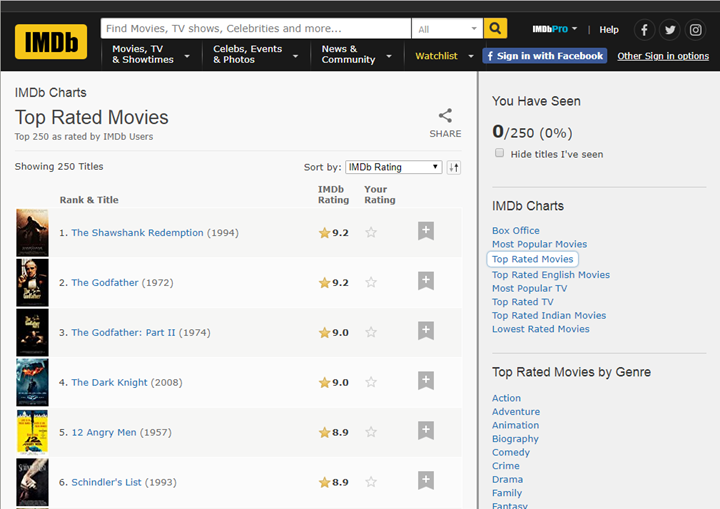• 点评数
• 点评分

• 电影A，十个人看过，全部评分均为10分
• 电影B，十万个人看过，评分平均值为8分

IMDB排名算法采用的是贝叶斯定理确定的其分值。在了解贝叶斯定理前，我们想要来理清一些概念。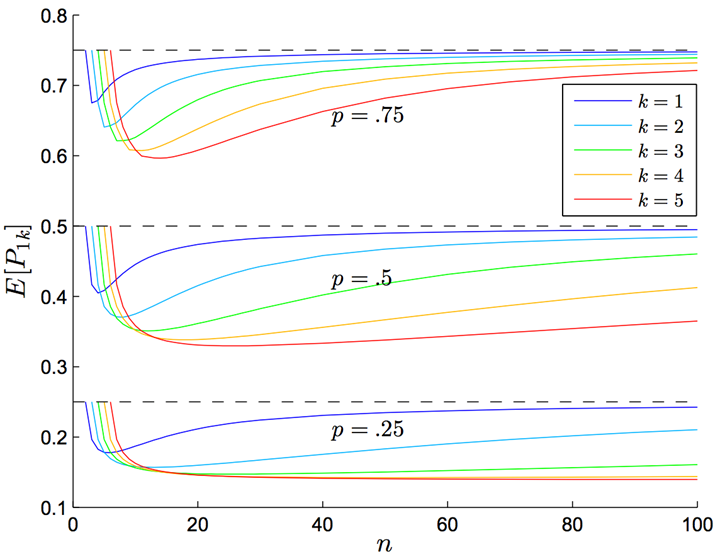$$P(A|B) = \frac{P(A)P(B|A)}{P(B)}$$

$$P(B)=P(A,B)+P(A^C,B)=P(B|A)P(A)+P(B|A^C)P(A^C)$$

IMDB排名公式

$$WR=\frac{v}{v+m}R+\frac{m}{v+m}C$$

• WR， 加权得分
• v，该电影的投票人数
• m，排名前 250 名的电影的最低投票数（人为设定的，目前好像是25000）
• R，该电影的用户投票的平均得分
• C，所有电影的平均得分（现在为9）

• IMDB为每部电影增加了25000张选票，并且这些选票的评分都为9。
• 假设所有电影都至少有25000张选票，那么就都具备了进入前250名的评选条件
• 假设这25000张选票的评分是所有电影的平均得分（即假设这部电影具有平均水准）
• 用现有的观众投票进行修正
• 长期来看，v/(v+m)这部分的权重将越来越大，得分将慢慢接近真实情况。

IMDB电影排名算法的缺陷：

• 新上映的电影短时间内评分上不去（点评量达不到要求）
• 能进入TOP 250的肯定是好电影，不是所有的好电影都能进入TOP 250

$$\bar{x}=\frac{C\times m+\sum_{i=1}^{n}x_i}{n+C}$$

• C，投票人数扩展的规模，是一个自行设定的常数，与整个网站的总体用户人数有关，可以等于每个项目的平均投票数。
• n，该项目的现有投票人数。
• x，该项目的每张选票的值。
• m，总体平均分，即整个网站所有选票的算术平均值。

“贝叶斯平均”也有缺点，主要问题是它假设用户的投票是正态分布。比如，电影A有 10 个观众评分，5个为五星，5个为一星；电影B也有 10 个观众评分，都给了三星。这两部电影的平均得分（无论是算术平均，还是贝叶斯平均）都是三星，但是电影A可能比电影B更值得看。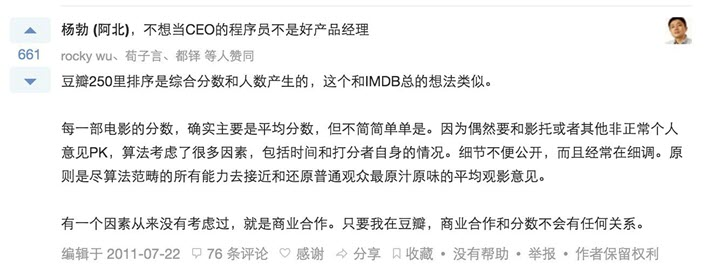## StackOverflow

Stack Overflow是一个专门针对程序员的问答网站，它能解决代码开发中遇到的很多问题。StackOverflow的排序共分为两类，1个是问题排序，1个是答案排序。这里主要介绍的是关于热门问题的排序。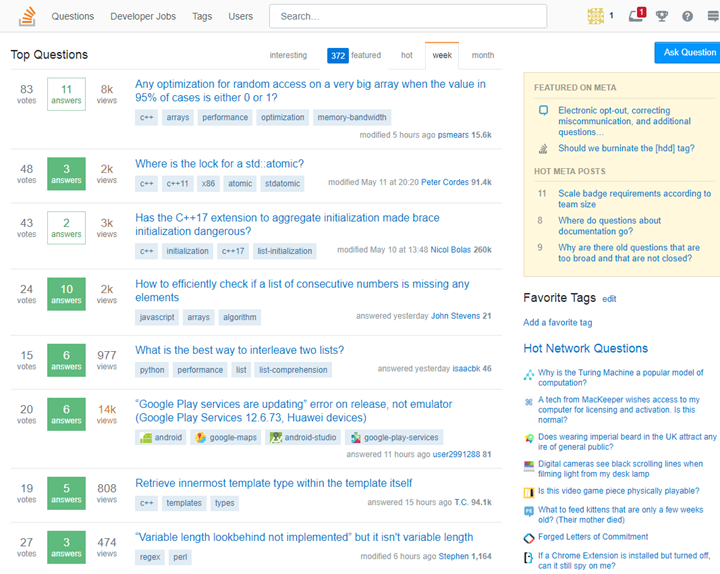StackOverflow在开始设计热门排序规则时考虑的因素：

• 问题的投票数，StackOverflow允许用户投反对票，所以这里可以使用绝对投票数，即正面票-负面票数量。绝对数越高问题越热门。
• 答案的投票数，即是否存在一个被大量认可的答案。这里存在两种情况，被提问者认可或被其他访问者投票。多少的投票量可以认为是问题答案被认可也是需要考虑的问题。
• 问题的浏览量，或是有效浏览量，有效浏览量可以建立一个停留时间的阀值去衡量。浏览的越多则越热门。
• 问题的答案数，理论上说答案越多则问题的越热门，但这也并不绝对，有些好的问答可能只有一个好的答案。
• 问题的提问时间和问题的最近回答时间，问题的受欢迎程度应该是随时间变长而变得不热门。
• 提问者的声望和回答者的声望，声望越高的问题肯定质量越到，越值得去推荐。

$$\frac{(\log_{10}Qviews)\times 4+\frac{Qanswers\times Qscore}{5}+sum(Ascores)}{((Qage+1)-(\frac{Qage-Qupdated}{2}))^{1.5}} = \frac{(\log_{10}Qviews)\times 4+\frac{Qanswers\times Qscore}{5}+sum(Ascores)}{(1+\frac{Qage}{2}+\frac{Qupdated}{2})^{1.5}}$$

Qviews（问题的浏览次数） log(Qviews)*4

Ascores（回答得分） sum(Ascores)

Qage（距离问题发表的时间）和 Qupdated（距离最后一个回答的时间） ((Qage+1) – ((Qage – Qupdated)/2)) ^ 1.5

Qage 和 Qupdated 的单位都是小时。如果一个问题的存在时间越久，或者距离上一次回答的时间越久，Qage 和 Qupdated 的值就相应增大。也就是说，随着时间流逝，这两个值都会越变越大，导致分母增大，因此总得分会越来越小。

import time, math

Qage = round((time.time() - date_ask) / 3600)
Qupdated = round((time.time() - date_active) / 3600)
return (math.log10(Qviews) * 4 + Qanswers * Qscore / 5 + Ascore) / (pow((Qage + 1) - (Qage - Qupdated) / 2, 1.5))


Stack Overflow 热点问题的排名，与参与度（Qviews 和 Qanswers）和质量（Qscore 和 Ascores）成正比，与时间（Qage 和 Qupdated）成反比。以下为个人思考点：

• 问题的质量与提问者的声望无正相关系
• 问题的答案与回答者的声望无正相关系
• 无名小辈也可以有精彩的问题与答案

$$\log_{10}{x}=\log_{b}{x}\Rightarrow b=\sqrt{10}=1.78$$

• 技术类问答，非开放性问题，往往答案只有一个，提问者的需求也仅仅需要一个答案
• Qscore = 赞成票-反对票，如果这个问题存在较大的争议，赞成票与反对票接近，是否是一个热门的问题？

• Qstatus：是否有答案，没有为0，有为1
• Qupvote：赞成票数量（考虑到Stackoverflow可能没有存赞成票数，用Qscore也可以）

• 问题A：有1个答案，投票数为10
• 问题B：有4个答案，投票数为4，3，2，1
• 问题C：有2个答案，投票数为10，-5

• 为什么要+1？防止问题刚提交时，公式中分母为0
• 为什么要÷2？把2小时作为最小的时间粒度单位
• 为什么要去5次方？添加“重力加速度”，提升曲线的陡度

• 发布时间越久，分值越低
• 回答时间越久，分值越低

## SegmentFault

SegmentFault 参考了Stack Overflow的热门算法设置了自己的排序算法，具体排序算法如下：

$$\frac{\lg(views)*4+recommendScore+collectScore+\ln(articleComments)}{(1+\frac{age}{2}+\frac{update}{2})^i}$$

• views：浏览量，对浏览量做了一次去对数处理，主要是为了防止某些浏览量较大的文章异军突起，待在榜单迟迟不动。
• recommendScore：文章的推荐数，直接加和到分子中，作为文章热门程度的考虑因素。
• collectScore：文章的收藏数，直接加和到分子中，作为文章热门程度的考虑因素。
• (1 + age/2 + update/2)^i：分母是对时间因子的考虑，宏观上来看，就是文章热度和创建时间成反比。细节上，做成了个指数函数，可以通过对 i 变量的调控来改变时间因子在对热度的影响。
• age：内容发布时间
• update：内容最后更新时间（所有时间值单位均为 h/3600）
• i：重力因子，取值的大小会直接决定热门排序（后面将介绍这点）

$$\frac{\lg(views)*4+\frac{sum(Qanswers * answerScores + Qscore)}{5}+ln(commentScore)}{(1+\frac{age}{2}+\frac{update}{2})^i}$$

• update：该问题下答案的最新更新时间

• 日热门的主要目的就是突出最近一天内的热门内容，更方便于内容被大家看到，文章快速地形成讨论、受关注的问题尽快得到解决；
• 周热门的主要目的很明确，就是突出过去一周内的热门内容，同时，给新产生的优秀内容机会，让其有机会进入热门列表；
• 月热门同周热门目的一样，但更需要给新内容进入列表的机会，以让内容经常更新。

• S是总得分指标
• t为时间量
• i是变量重力因子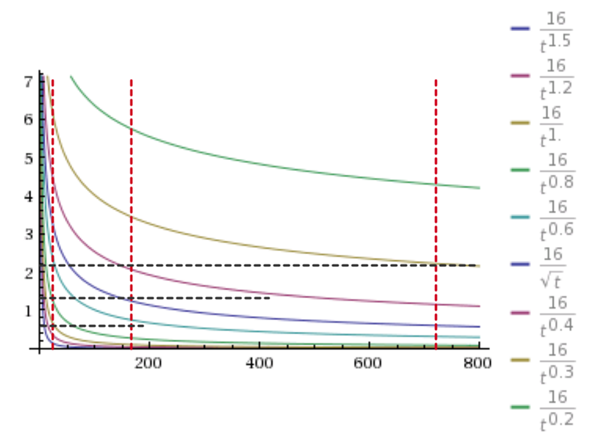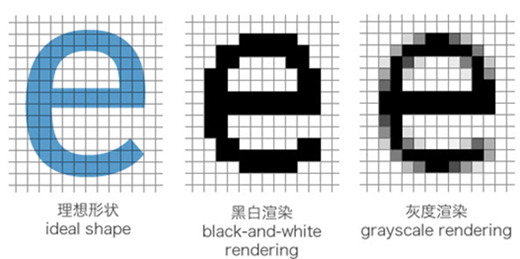##### 网站与APP开发中的字体设置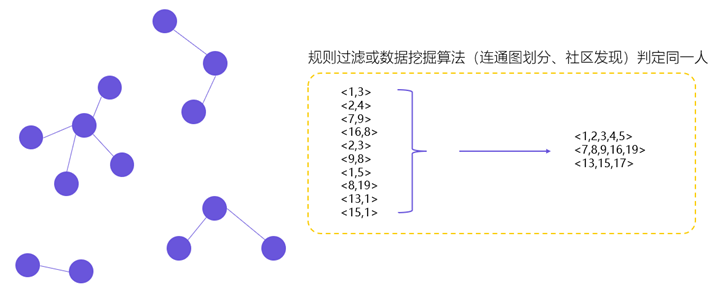##### 用户体系搭建之ID-Mapping